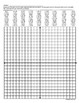# Parabolic Curve Graph Art ActivitySubject
Resource Type
File Type
PDF (341 KB|4 pages)
Standards
\$2.00
Also included in:
1. These print and go activities require students to find and graph ordered pairs on a coordinate plane graph. Ordered pairs include integers ranging from 12 to -12 and includes all 4 quadrants. Students use a ruler or straight edge to connect points in order to create this fun parabolic curve using al
\$6.00
\$4.20
Save \$1.80
• Product Description
• Standards

This print and go activity requires students to find and graph ordered pairs on a coordinate plane graph. Ordered pairs include integers ranging from 12 to -12 and includes all 4 quadrants. Students use a ruler or straight edge to connect points in order to create this fun parabolic curve using all straight lines. Such a cool idea to explore!

More Fun with Graphing Activities:

Parabolic Curves Version 2

Find and position integers and other rational numbers on a horizontal or vertical number line diagram; find and position pairs of integers and other rational numbers on a coordinate plane.
Understand a rational number as a point on the number line. Extend number line diagrams and coordinate axes familiar from previous grades to represent points on the line and in the plane with negative number coordinates.
Total Pages
4 pages